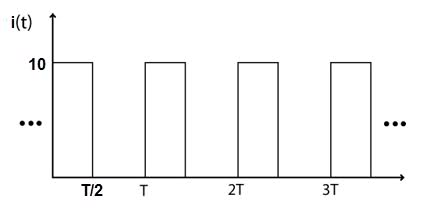# Energy waveform

From the given waveform (of current), sketch the energy from t = 0 to t = 2T. Given: R = 10 Ohms, i = 10 AmpsI'm having trouble with this even though it's probably really easy.
I know WR = ∫Ri2(t) dt
so for one period, for example, I have
∫10(102) dt with limits of integration from 0 to T/2
= 1000 t / t goes from 0 to T/2
= 500T
i believe this is a line with slope 500 going from 0 to T/2..but i'm not sure what i do for the other values of t
when i graph it should I just graph 1000t and plug in values for t?

rude man
Homework Helper
Gold Member
Energy = time integral of power.

So you're doing just fine up to T/2.

Question to you: given the above, what should the curve look like between T2 and T?

And when the second pulse starts, until 3T/2?
And from 3T/2 to 2T?

i would assume it just repeats, and from T/2 to T it would be zero and again from 5T/2 to 3T. but would it be somewhat like a sawtooth wave?

rude man
Homework Helper
Gold Member
Why would it be zero from T/2 to T? Where did the energy go?

BTW the problem doesn't ask you to go beyond 2T so I wouldn't.

NascentOxygen
Staff Emeritus
from T/2 to T it would be zero
When I is zero then additional energy dissipated as heat in the resistor would be zero. Note: additional energy during T/2 -> T is zero.

When I is zero then additional energy dissipated as heat in the resistor would be zero. Note: additional energy during T/2 -> T is zero.

why additional? what other energy is there? or where does it come from?
i only know of energy being equal to ∫p dt = ∫ Ri2(t) dt, and isn't p 0 from T/2 to T?

rude man
Homework Helper
Gold Member
Magnifik -please - for the last time - the energy you built up from 0 to T/2 has not disasppeared, so your graph just HAS to show it!!! Energy cannot be created or destroyed!!!

Yes - P is zero, but E is not, it's what it was at t = T/2 -.

NascentOxygen
Staff Emeritus
It's a resistor, so we are not talking about some vague concept or interplay of various energy forms. We are talking HEAT. Total heat energy. It's a graph that keeps increasing (never dropping) because it is a plot summing total heat energy since time t=0.

i'm still confused as to what the graph would look like.
is the total energy delivered over one period 500T? ... i think i just understood why T/2 to T is not 0 with that question

Last edited:
rude man
Homework Helper
Gold Member
i'm still confused as to what the graph would look like.
is the total energy delivered over one period 500T? ... i think i just understood why T/2 to T is not 0 with that question

Let me try an analogy. You have a 100W light bulb. You turn it on for 1/2 hour and then turn it off for the next half hour.

Now graph what the watt-hour meter reads during the 1 hour time period.

Let me try an analogy. You have a 100W light bulb. You turn it on for 1/2 hour and then turn it off for the next half hour.

Now graph what the watt-hour meter reads during the 1 hour time period.

It would be 50 watt-hour for the hour long time period. then the next hour would be 50 again so total would be 100, etc., etc.
if i plotted it over time, it would be a line with positive slope, yes?

rude man
Homework Helper
Gold Member
It would be 50 watt-hour for the hour long time period. then the next hour would be 50 again so total would be 100, etc., etc.
if i plotted it over time, it would be a line with positive slope, yes?

I asked for just the 1 hour interval.

No, it would read 100t watt-hours during the first 30 minutes and 50 watt-hrs after that until 1 hr. t is in hours.

I asked for just the 1 hour interval.

No, it would read 100t watt-hours during the first 30 minutes and 50 watt-hrs after that until 1 hr. t is in hours.

Woops, misread your question. I thought you meant it was already on for 30 minutes and then off for the next 30 minutes so the power was like a pulse train.

I thought for energy
It's a graph that keeps increasing (never dropping) because it is a plot summing total heat energy since time t=0.

Last edited:
rude man
Homework Helper
Gold Member
Woops, misread your question. I thought you meant it was already on for 30 minutes and then off for the next 30 minutes so the power was like a pulse train.

That is what I meant. The bulb is on for 0< t < 30 min. and off for 30 < t < 1 hr.

I thought for energy
It's a graph that keeps increasing (never dropping) because it is a plot summing total heat energy since time t=0.

Now you're cooking with gas - or whatever your electric utility burns! :-)